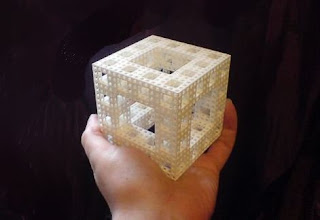## Saturday, 26 March 2011

### A Tilable Space-filling Fractal SpongeA fractal, tileable sponge ("Alt.Fractals", p.61)

Okay, so this one looks like a "stretch" version of the conventional Menger Sponge, using a 4×4×4 grid instead of 3×3×3 .. and it is ... but it's a bit more than that. It's not just an arbitrary variation on a math standard.

It's a space-filling solid.

Space-filling solids are kinda rare. A cube will tile 3D space (obviously), but the other Platonic Solids won't, at least, not individually. A small group of other regular-ish solids have the property, like the truncated octahedron (which has a mix of square and hexagonal sides), and the rhombic dodecahedron (which has twelve identical diamond-shaped faces), but you won't normally find any mentions of space-filling solids that are also fractals.

Let's look at this shape further. It's constructed by taking a cube and dividing it into a 4×4×4 grid of smaller cubes, and deleting central 2×2 columns through the centre of each face (and then repeating, ad infinitum).
The 4×4×4 grid gives us 64 smaller cubes, so when we then delete four cubes per face, and eight from the centre, we're eliminating 6×4+8=32 cubes, out of the original 64. Every time we cut a new generation of holes, the remaining volume of the sponge halves.

Now, how it tiles.

For the "zeroth" generation, we just have a simple cube, which will (obviously) tile an infinite 3D space as an array of (infinity^3) individual pieces. Now cut the first generation of holes into each piece. The holes punch an intersecting series of aligned 2×2 tunnels through the array, and where the 1×1 edge-columns of adjacent cubes touch, they form an intersecting series of 2×2 beams. The networks of columns and tunnels have the same shape, and we've already established that they have the same volume, so after one generation, we can take our infinite array, make a copy of it, and fit the copy exactly into the holes of the original to make it totally solid.

If we then take our resulting solid "dual" array, and cut the second-generation holes into each individual piece, the volume again halves, the new holes in both arrays again line up, and again we have identical networks of solids and spaces. Again, we can fit an identical offset copy of the original exactly into the spaces.

This goes on forever. For one of these sponges with n generations of holes, you can tile space solidly with n^2 overlapping copies of the sponge coexisting in the same space, so for the third-generation sponge shown in the photograph, you can interleave eight of them together.

For the "perfect" version of the sponge, with an infinite number of holes, you can tile space solidly with a block assembled from (2^infinity) overlapping "same-size" copies existing in the same space. Strange, but true.

So, it's Challenge Time! Can anyone find any other fractal solids that will completely tile space at a fixed size?